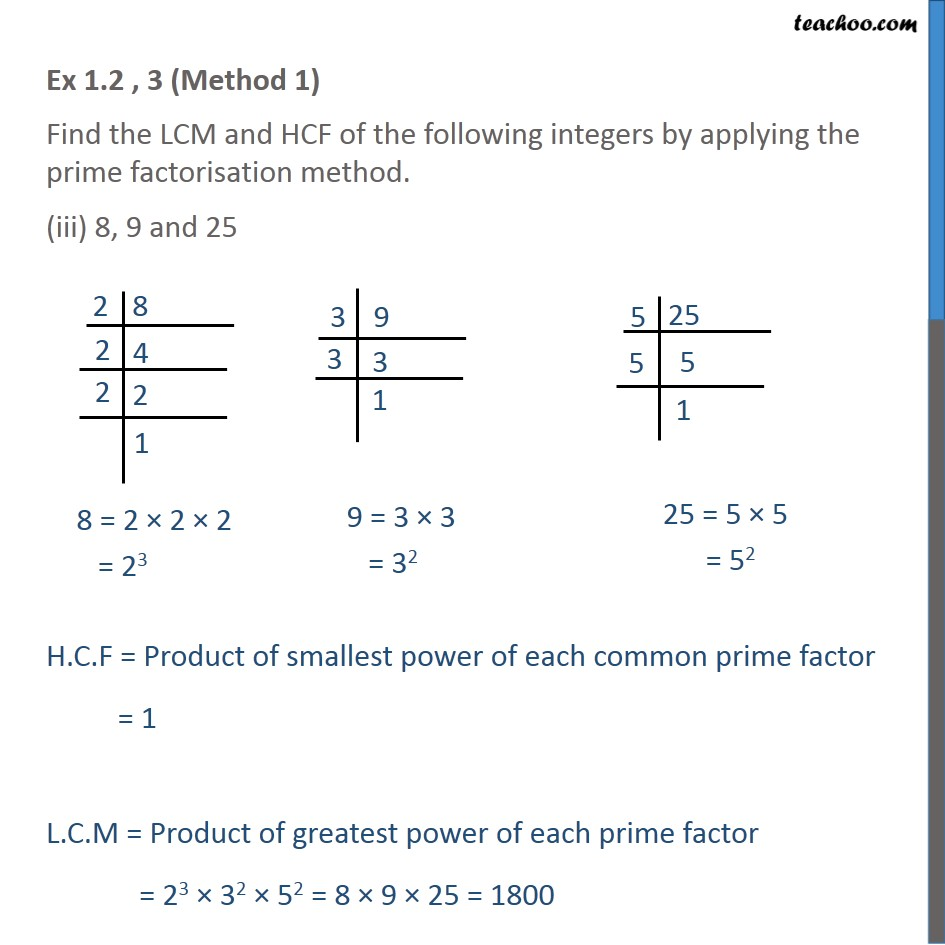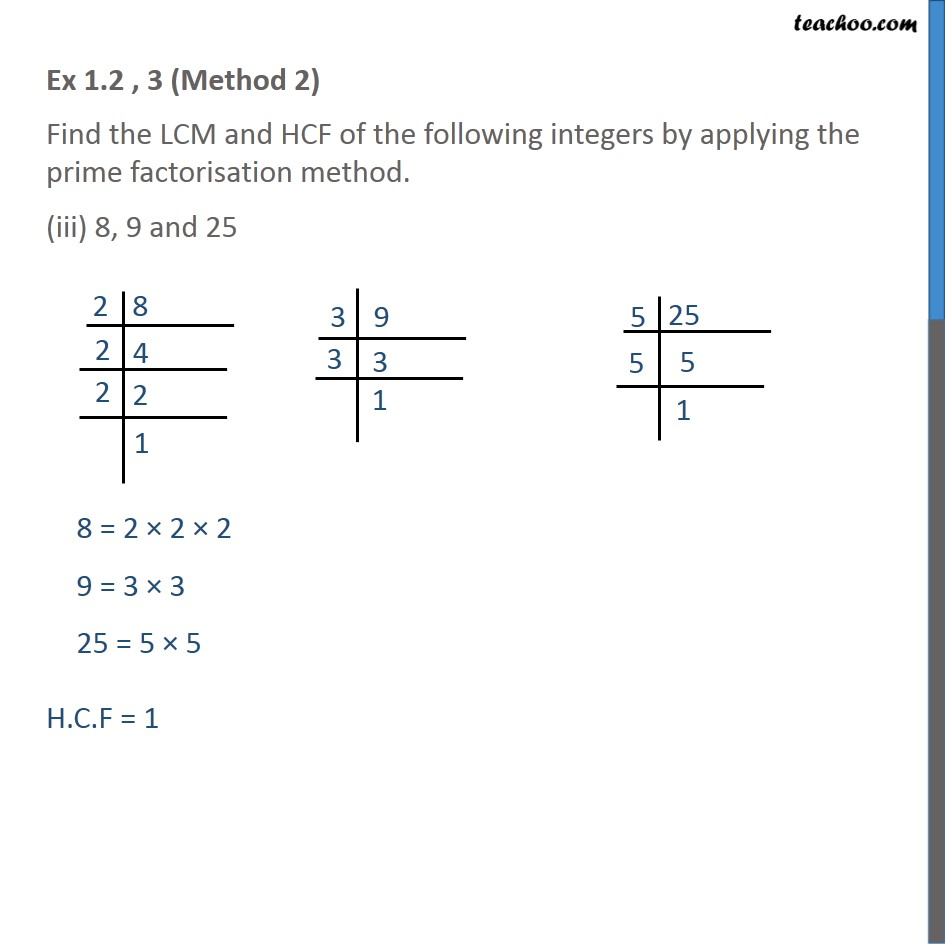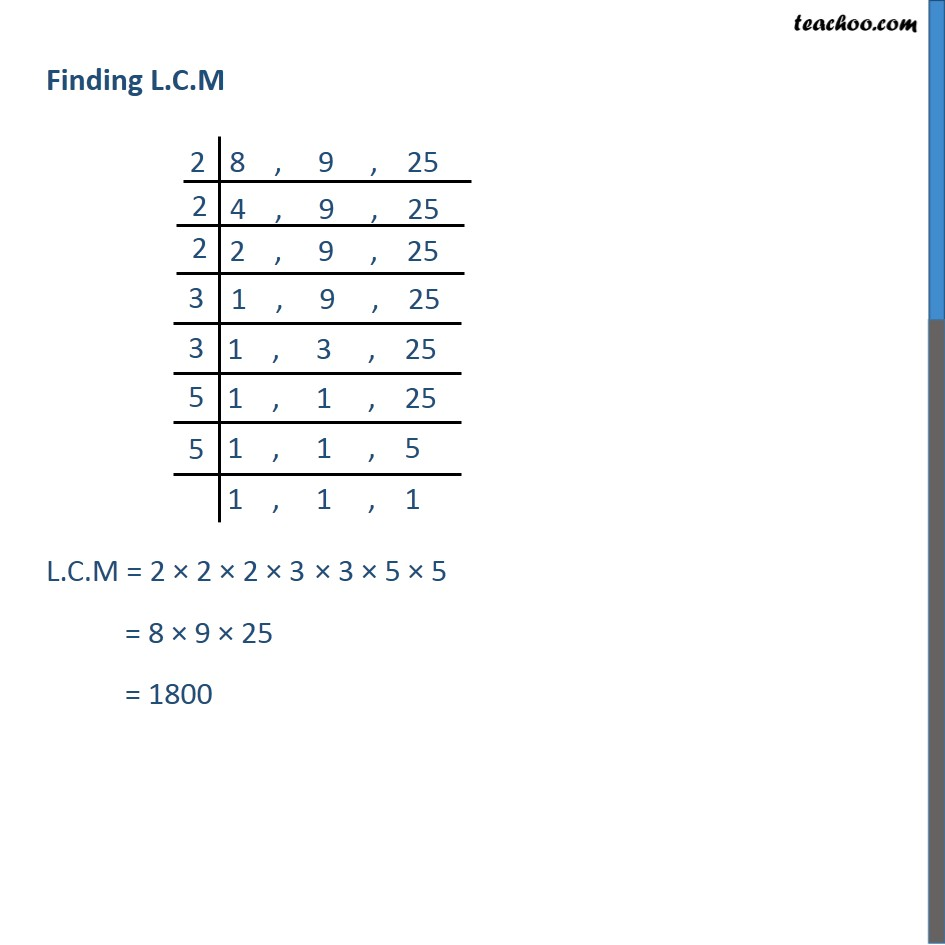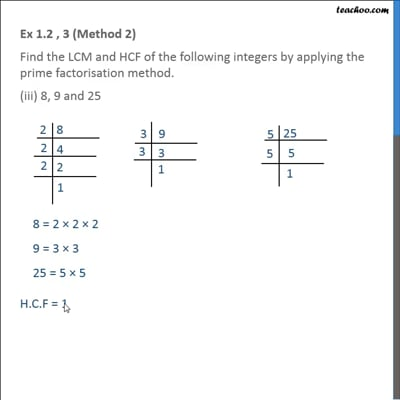Ex 1.2

Chapter 1 Class 10 Real Numbers
Serial order wiseThis video is only available for Teachoo black users

Introducing your new favourite teacher - Teachoo Black, at only ₹83 per month

### Transcript

Ex 1.2 , 3 (Method 1) Find the LCM and HCF of the following integers by applying the prime factorisation method. (iii) 8, 9 and 25 8 = 2 × 2 × 2 = 23 9 = 3 × 3 = 32 25 = 5 × 5 = 52 H.C.F = Product of smallest power of each common prime factor = 1 L.C.M = Product of greatest power of each prime factor = 23 × 32 × 52 = 8 × 9 × 25 = 1800 Ex 1.2 , 3 (Method 2) Find the LCM and HCF of the following integers by applying the prime factorisation method. (iii) 8, 9 and 25 8 = 2 × 2 × 2 9 = 3 × 3 25 = 5 × 5 H.C.F = 1 L.C.M = 2 × 2 × 2 × 3 × 3 × 5 × 5 = 8 × 9 × 25 = 1800